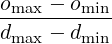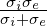### 8.3 Session file configuration

#### 8.3.1 Solver Info

• `Eqtype` Specifies the PDE system to solve. The following values are supported:
• `Monodomain`: solve the monodomain equation.
• `BidomainRoth`: solve the bidomain equations using the Roth formulation.
• `CellModel` Specifies the cell model to use. Available cell models are

 Value Description No. of Var. Ref. `AlievPanfilov` Phenomological 1  `CourtemancheRamirezNattel98` Human atrial 20  `FitzHughNagumo` `Fox02` `LuoRudy91` Mammalian ventricular 7  `PanditGilesDemir03` `TenTusscher06` Human ventricular 18  `Winslow99`
• `Projection` Specifies the Galerkin projection type to use. Only `Continuous` has been extensively tested.
• `TimeIntegrationMethod` Specifies the time integration scheme to use for advancing the PDE system. This must be an IMEX scheme. Suitable choices are: `IMEXOrder1`, `IMEXOrder2`, `IMEXOrder3`, `IMEXdirk_3_4_3`. The cell model state variables are time advanced using Forward Euler for the ion concentrations, and Rush-Larsen for the cell model gating variables.
• `DiffusionAdvancement` Specifies whether the diffusion is handled implicitly or explicitly in the time integration scheme. The current code only supports `Implicit` integration of the diffusion term. The cell model is always integrated explicitly.

#### 8.3.2 Parameters

The following parameters can be specified in the `PARAMETERS` section of the session file. Example values are taken from .

• `Chi` sets the surface-to-volume ratio (Units: mm-1).
Example: χ = 140mm-1
• `Cm` sets the specific membrane capacitance (Units: μFmm-2).
Example: Cm = 0.01μFmm-2
• `Substeps` sets the number of substeps taken in time integrating the cell model for each PDE timestep.
Example: 4
• `d_min`, `d_max`, `o_min`, `o_max` specifies a bijective map to assign conductivity values σ to intensity values μ when using the `IsotropicConductivity` function. The intensity map is first thresholded to the range [dmin,dmax] and then the conductivity is calculated as  σ =(1 - μ) + omin

#### 8.3.3 Functions

The following functions can be specified inside the `CONDITIONS` section of the session file. If both are specified, the effect is multiplicative. Example values are taken from .

• `IsotropicConductivity` specifies the conductivity σ of the tissue.
Example: σ = 0.13341 mSmm-1, based on σ =,  σi = 0.17,σe = 0.62mSmm-1

The variable name to use is `intensity` since the conductivity may be derived from late-Gadolinium enhanced MRA imaging. Example specifications are

1<E VAR="intensity" VALUE="0.13341" />
2<F VAR="intensity" FILE="scarmap.con" />

where `scarmap.con` is a Nektar++ field file containing a variable `intensity` describing the conductivity across the domain.

• `AnisotropicConductivity` specifies the conductivity σ of the tissue.

#### 8.3.4 Filters

The following filters are supported exclusively for the cardiac EP solver. Further filters from section 3.4 are also available for this solver.

• `Benchmark` (section 3.4.3)
• `CellHistoryPoints` (section 3.4.4)
• `CheckpointCellModel` (section 3.4.5)
• `Electrogram` (section 3.4.7)

#### 8.3.5 Stimuli

Electrophysiological propagaion is initiated through the stimulus current Iion. The `STIMULI` section describes one or more regions of stimulus and the time-dependent protocol with which they are applied.

1<STIMULI>
2  ...
3</STIMULI>

A number of stimulus types are available

##### 8.3.5.1 Stimulus types
• `StimulusRect` stimulates a cuboid-shaped region of the domain, specified by two coordinates (x1,y1,z1) and (x2,y2,z2). An additional parameter specifies the "smoothness" of the boundaries of the region; higher values produce a sharper boundary. Finally, the maximum strength of the stimulus current is specified in μA∕mm3
1<STIMULUS TYPE="StimulusRect" ID="0">
2  <p_x1> -15.24 </p_x1>
3  <p_y1>  14.02 </p_y1>
4  <p_z1>   6.87 </p_z1>
5  <p_x2>  12.23 </p_x2>
6  <p_y2>  16.56 </p_y2>
7  <p_z2>   8.88 </p_z2>
8  <p_is> 100.00 </p_is>
9  <p_strength> 50.0 </p_strength>
10</STIMULUS>
• `StimulusCirc` stimulates a spherical region of the domain, as specified by a centre and radius. The smoothness and strength parameters are also specified as for ‘StimulusRect‘.
1<STIMULUS TYPE="StimulusCirc" ID="0">
2  <p_x1> -15.24 </p_x1>
3  <p_y1>  14.02 </p_y1>
4  <p_z1>   6.87 </p_z1>
5  <p_r1>  12.23 </p_r1>
6  <p_is> 100.00 </p_is>
7  <p_strength> 50.0 </p_strength>
8</STIMULUS>

##### 8.3.5.2 Protocols

A protocol specifies the time-dependent function indicating the strength of the stimulus and one such `PROTOCOL` section should be included within each `STIMULUS`. This can be expressed as one of:

• `ProtocolSingle` a single stimulus is applied at a given start time and for a given duration
1<PROTOCOL TYPE="ProtocolSingle">
2  <START>    0.0   </START>
3  <DURATION> 2.0   </DURATION>
4</PROTOCOL>
• `ProtocolS1` a train of pulses of fixed duration applied at a given start time and with a given cycle length.
1<PROTOCOL TYPE="ProtocolS1">
2  <START>    0.0   </START>
3  <DURATION> 2.0   </DURATION>
4  <S1CYCLELENGTH> 300.0 </S1CYCLELENGTH>
5  <NUM_S1>   5     </NUM_S1>
6</PROTOCOL>
• `ProtocolS1S2` same as ‘ProtocolS1‘ except with an additional single pulse applied at a different cycle length at the end of the train of S1 pulses.
1<PROTOCOL TYPE="ProtocolS1S2">
2  <START>    0.0   </START>
3  <DURATION> 2.0   </DURATION>
4  <S1CYCLELENGTH> 300.0 </S1CYCLELENGTH>
5  <NUM_S1>   5     </NUM_S1>
6  <S2CYCLELENGTH> 100.0 </S2CYCLELENGTH>
7</PROTOCOL>